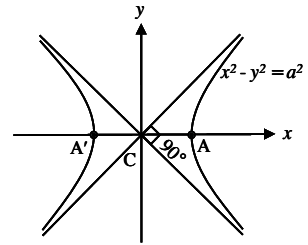# Rectangular Hyperbola

A hyperbola is said to be a rectangular hyperbola if its asymptotes are at right angles.

The angle between the asymptotes is given by 2 tan−1 (b/a).

b/a = tan 45° = 1

a = b

The equation of rectangular hyperbola is

x2 − y2 = a2

The combined equation of the asymptotes is x2 − y2 = 0. The separate equations are x − y = 0 and x + y = 0. i.e., x = y and x = −y. The transverse axis is y = 0, conjugate axis is x = 0.